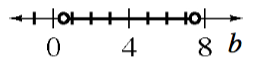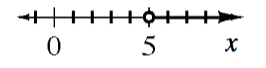### Home > INT2 > Chapter 9 > Lesson 9.3.1 > Problem9-87

9-87.

Solve each inequality below and graph your solution on a number line.

1. $(b - 4)^2 < 12$

Change the inequality to an equation and solve the equation. Use the solution(s) to the equation to divide the number line into regions. Test a point in each region to determine if the region is part of the solution or not.

$≈ 0.54 < b < ≈ 7.46$1. $(x + 3)^2 > 4$

Refer to part (a).

1. $2(x - 3) > 4$

Divide both sides by $2$ before trying to solve the inequality.

$x > 5$